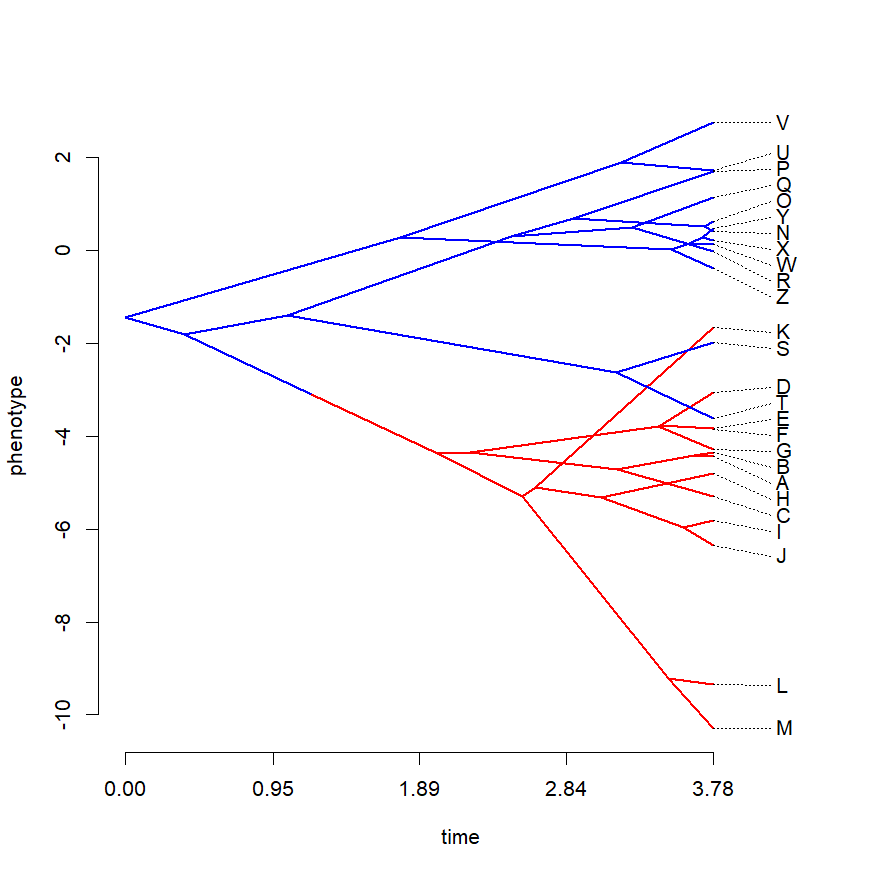## Thursday, September 5, 2019

### `logLik` method for `brownie.lite`

I just pushed a small update to phytools giving the popular `brownie.lite` function a `logLik` S3 method.

`brownie.lite` fits a multi-rate Brownian motion model originally developed by Brian O'Meara et al. (2006).

Here, I'll show how it can be used in combination with the generic `AIC` method to do model selection or to compute model weights:

``````library(phytools)
tree
``````
``````##
## Phylogenetic tree with 26 tips and 25 internal nodes.
##
## Tip labels:
##  A, B, C, D, E, F, ...
##
## The tree includes a mapped, 2-state discrete character with states:
##  a, b
##
## Rooted; includes branch lengths.
``````
``````x
``````
``````##            A            B            C            D            E
##  -4.42905986  -4.33868707  -5.30046141  -3.05157557  -3.82136084
##            F            G            H            I            J
##  -3.84278970  -4.28031068  -4.79455901  -5.81169561  -6.34045086
##            K            L            M            N            O
##  -1.65886570  -9.33620130 -10.28889806   0.41905850   0.62457524
##            P            Q            R            S            T
##   1.69227697   1.14144175  -0.02283264  -1.98316222  -3.61491689
##            U            V            W            X            Y
##   1.71643871   2.75486352   0.13485761   0.21096964   0.47606198
##            Z
##  -0.38421995
``````
``````cols<-setNames(c("blue","red"),c("a","b"))
phenogram(tree,x,colors=cols)
````````````##            1            2            3            4            5
##  -5.01570904  -4.67036911  -5.70806984  -2.94258054  -3.63500326
##            6            7            8            9           10
##  -3.98014777  -4.32528265  -5.36044268  -6.05326441  -6.58088057
##           11           12           13           14           15
##  -1.76580067  -9.37186885 -10.28889806   0.36848651   1.05920932
##           16           17           18           19           20
##   1.74996436   1.40415384  -0.66609199  -2.11198563  -3.28891866
##           21           22           23           24           25
##   2.09536923   2.75486352  -0.32092830   0.02392802   0.71404775
##           26
##  -1.01180397
``````
``````## fit two-rate model using brownie.lite
fit<-brownie.lite(tree,x)
fit
``````
``````## ML single-rate model:
##  s^2 se  a   k   logL
## value    2.2226  0.6164  -1.4325 2   -45.13
##
## ML multi-rate model:
##  s^2(a)  se(a)   s^2(b)  se(b)   a   k   logL
## value    1.0376  0.4312  3.6835  1.5578  -0.6666 3   -43.2547
##
## P-value (based on X^2): 0.0528
##
## R thinks it has found the ML solution.
``````
``````liks<-logLik(fit)
liks
``````
``````## single-rate  multi-rate
##   -45.12998   -43.25468
## attr(,"df")
##  2 3
``````
``````AIC<-setNames(AIC(fit),names(liks))
AIC
``````
``````## single-rate  multi-rate
##    94.25996    92.50935
``````
``````aic.w(AIC)
``````
``````## single-rate  multi-rate
##    0.294152    0.705848
``````

The data were simulated as follows:

``````set.seed(1)
tree<-pbtree(n=26,tip.label=LETTERS)
tree<-paintSubTree(tree,29,"b","a",stem=0.5)
x<-sim.rates(tree,setNames(c(1,2.5),letters[1:2]))
``````